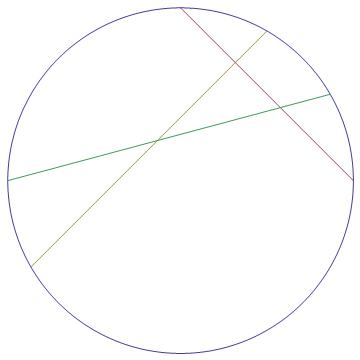# Pizza Cutting Puzzle!

Geometry Level 5

A 12 inch diameter round pizzahas been sliced by 3 straight cuts, leaving a right triangle in the middle as shown hereThe edge of the pizza has been cut into 6 arcs, one each of the following, in degrees, totaling 360 degrees:

${15, 30, 45, 60, 90, 120}$

but not necessarily in that order. The maximum area, in square inches, this right triangle piece in the middle can have can be expressed as

$\dfrac { 1 }{ a } (b+c\sqrt { 2 } +d\sqrt { 3 } +e\sqrt { 6 } )$

where $a, b, c, d, e$ are integers (which may be negative). Find $a+b+c+d+e$.

Integer $a$ is positive. Do not rely on diagram to guess arcs in degrees.

×